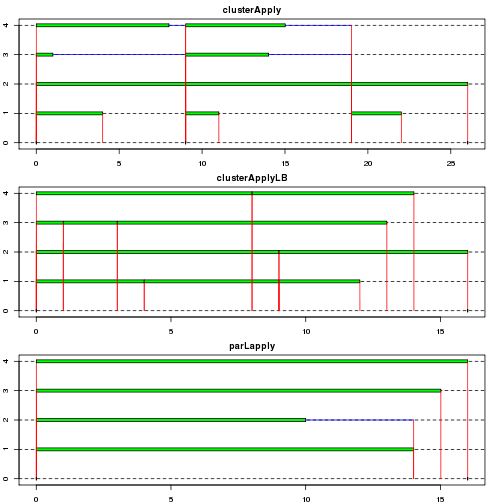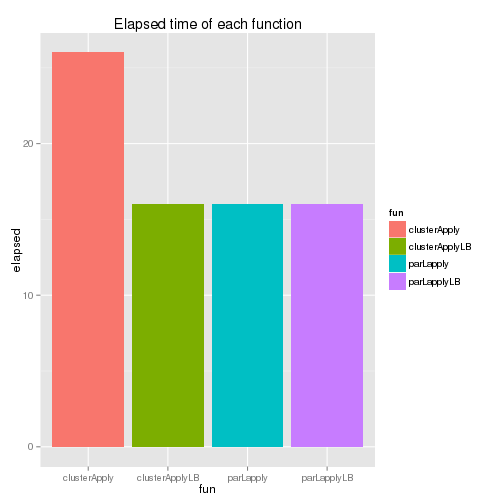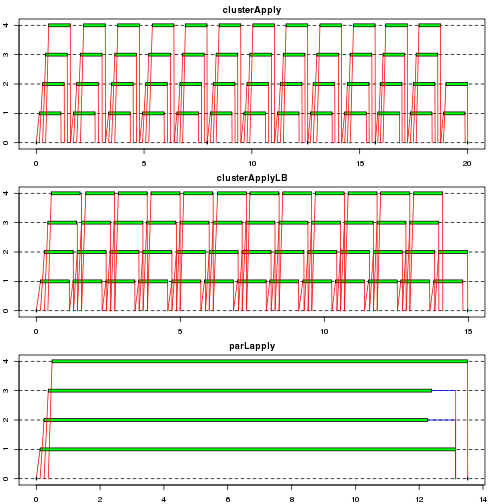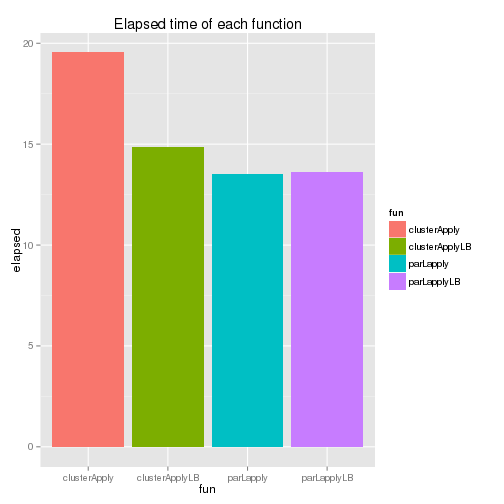Lack of multi-threading and memory limitation are two outstanding weaknesses of base R. In fact, however, if the size of data is not so large that it can be read in RAM, the former would be relatively easily handled by parallel processing, provided that multiple processors are equipped. This article introduces to a way of implementing parallel processing on a single machine using the snow and parallel packages - the examples are largely based on McCallum and Weston (2012).

The snow and multicore are two of the packages for parallel processing and the parallel package, which has been included in the base R distribution by CRAN (since R 2.14.0), provides functions of both the packages (and more). Only the functions based on the snow package are covered in the article.

The functions are similar to lapply() and they just have an additional argument for a cluster object (cl). The following 4 functions will be compared.

• clusterApply(cl, x, fun, ...)
• pushes tasks to workers by distributing x elements
• clusterApplyLB(cl, x, fun, ...)
• efficient if some tasks take longer or some workers are slower
• parLapply(cl, x, fun, ...)
• clusterApply + scheduling tasks by splitting x given clusters
• docall(c, clusterApply(cl, splitList(x, length(cl)), lapply, fun, …))
• parLapplyLB(cl = NULL, X, fun, ...)
• available only in the parallel package

Let’s get started.

As the packages share the same function names, the following utility function is used to reset environment at the end of examples.

 1reset = function(package) {
3    detach(search()[grep(paste0("*",pkg),search())]
5           ,character.only=TRUE)
6  }
8  rm(list = ls()[ls()!="knitPost"]) # leave utility function
9  rm(list = ls()[ls()!="moveFigs"]) # leave utility function
10  par(mfrow=c(1,1),mar=c(5.1, 4.1, 4.1, 2.1)) # reset graphics parameters
11}


## Make and stop a cluster

In order to make a cluster, the socket transport is selected (type=“SOCK” or type=“PSOCK”) and the number of workers are set manually using the snow package (spec=4). In the parallel package, it can be detected by detectCores(). stopCluster() stops a cluster.

 1## make and stop cluster
2require(snow)
3spec = 4
4cl = makeCluster(spec, type="SOCK")
5stopCluster(cl)
6reset("snow")
7
8require(parallel)
9# number of workers can be detected
10# type: "PSOCK" or "FORK", default - "PSOCK"
11cl = makeCluster(detectCores())
12stopCluster(cl)
13reset("parallel")


## CASE I - load balancing matters

As mentioned earlier, load balancing can be important when some tasks take longer or some workers are slower. In this example, system sleep time is assigned randomly so as to compare how tasks are performed using snow.time() in the snow package and how long it takes by the functions using system.time().

 1# snow
2require(snow)
3set.seed(1237)
4sleep = sample(1:10,10)
5spec = 4
6cl = makeCluster(spec, type="SOCK")
7st = snow.time(clusterApply(cl, sleep, Sys.sleep))
8stLB = snow.time(clusterApplyLB(cl, sleep, Sys.sleep))
9stPL = snow.time(parLapply(cl, sleep, Sys.sleep))
10stopCluster(cl)
11par(mfrow=c(3,1),mar=rep(2,4))
12plot(st, title="clusterApply")
13plot(stLB, title="clusterApplyLB")
14plot(stPL, title="parLapply")Both clusterApplyLB() and parLapply() takes shorter than clusterApply(). The efficiency of the former is due to load balancing (pulling a task when necessary) while that of the latter is because of a lower number of I/O operations thanks to task scheduling, which allows a single I/O operation in a chunk (or split) - its benefit is more outstanding when one or more arguments are sent to workers as shown in the next example. The scheduling can be checked by clusterSplit().

1sleep

##    4  9  1  8  2 10  5  6  3  7

1clusterSplit(cl, sleep)

## []
##  4 9 1
##
## []
##  8 2
##
## []
##  10  5
##
## []
##  6 3 7

1# clear env
2reset("snow")


The above functions can also be executed using the parallel package and it provides an additional function (parLapplyLB()). As snow.time() is not available, their system time is compared below.

 1# parallel
2require(parallel)
3set.seed(1237)
4sleep = sample(1:10,10)
5cl = makeCluster(detectCores())
6st = system.time(clusterApply(cl, sleep, Sys.sleep))
7stLB = system.time(clusterApplyLB(cl, sleep, Sys.sleep))
8stPL = system.time(parLapply(cl, sleep, Sys.sleep))
9stPLB = system.time(parLapplyLB(cl, sleep, Sys.sleep))
10stopCluster(cl)
11sysTime = do.call("rbind",list(st,stLB,stPL,stPLB))
12sysTime = cbind(sysTime,data.frame(fun=c("clusterApply","clusterApplyLB"
13                                         ,"parLapply","parLapplyLB")))
14require(ggplot2)
15ggplot(data=sysTime, aes(x=fun,y=elapsed,fill=fun)) +
16  geom_bar(stat="identity") + ggtitle("Elapsed time of each function")1# clear env
2reset("parallel")


## CASE II - I/O operation matters

In this example, a case where an argument is sent to workers is considered. While the argument is passed to wokers once for each task by clusterApply() and clusterApplyLB(), it is sent to each chunk once by parLapply() and parLapplyLB(). Therefore the benefit of the latter group of functions can be outstanding in this example - it can be checked workers are idle inbetween in the first two plots while tasks are performed continuously in the last plot.

 1# snow
2require(snow)
3mat = matrix(0, 2000, 2000)
4sleep = rep(1,50)
5fcn = function(st, arg) Sys.sleep(st)
6spec = 4
7cl = makeCluster(spec, type="SOCK")
8st = snow.time(clusterApply(cl, sleep, fcn, arg=mat))
9stLB = snow.time(clusterApplyLB(cl, sleep, fcn, arg=mat))
10stPL = snow.time(parLapply(cl, sleep, fcn, arg=mat))
11stopCluster(cl)
12par(mfrow=c(3,1),mar=rep(2,4))
13plot(st, title="clusterApply")
14plot(stLB, title="clusterApplyLB")
15plot(stPL, title="parLapply")1# clear env
2reset("snow")


Although clusterApplyLB() has some improvement over clusterApply(), it is parLapply() which takes the least amount of time. Actually, for the snow package, McCallum and Weston (2012) recommends parLapply() and it’d be better to use parLapplyLB() if the parallel package is used. The elapsed time of each function is shown below - the last two functions’ elapsed time is identical as individual tasks are assumed to take exactly the same amount of time.

 1# parallel
2require(parallel)
3mat = matrix(0, 2000, 2000)
4sleep = rep(1,50)
5fcn = function(st, arg) Sys.sleep(st)
6cl = makeCluster(detectCores())
7st = system.time(clusterApply(cl, sleep, fcn, arg=mat))
8stLB = system.time(clusterApplyLB(cl, sleep, fcn, arg=mat))
9stPL = system.time(parLapply(cl, sleep, fcn, arg=mat))
10stPLB = system.time(parLapplyLB(cl, sleep, fcn, arg=mat))
11stopCluster(cl)
12sysTime = do.call("rbind",list(st,stLB,stPL,stPLB))
13sysTime = cbind(sysTime,data.frame(fun=c("clusterApply","clusterApplyLB"
14                                         ,"parLapply","parLapplyLB")))
15require(ggplot2)
16ggplot(data=sysTime, aes(x=fun,y=elapsed,fill=fun)) +
17  geom_bar(stat="identity") + ggtitle("Elapsed time of each function")1# clear env
2reset("parallel")


## Initialization of workers

Sometimes workers have to be initialized (eg loading a library) and two functions can be used: clusterEvalQ() and clusterCall(). While the former just executes an expression, it is possible to send a variable using the latter. Note that it is recommended to let an expression or a function return NULL in order not to receive unnecessary data from workers (McCallum and Weston (2012)). Only an example by the snow package is shown below.

 1# snow and parallel
2require(snow)
3spec = 4
4cl = makeCluster(spec, type="SOCK")
5# execute expression
6exp = clusterEvalQ(cl, { library(MASS); NULL })
7# execute expression + pass variables
8worker.init = function(arg) {
9  for(a in arg) library(a, character.only=TRUE)
10  NULL
11}
12expCall = clusterCall(cl, worker.init, arg=c("MASS","boot"))
13stopCluster(cl)
14
15# clear env
16reset("snow")


## Random number generation

In order to ensure that each worker has different random numbers, independent randome number streams have to be set up. In the snow package, either the L’Ecuyer’s random number generator by the rlecuyer package or the SPRNG generator by the rsprng are used in clusterSetupRNG(). Only the former is implemented in the parallel package in clusterSetRNGStream() and, as it uses its own algorithm, the rlecuyer package is not necessary. For reproducibility, a seed can be specified and, for the function in the snow package, a vector of six integers is necessary (eg seed=rep(1237,6)) while an integer value is required for the function in the parallel package (eg iseed=1237). Each of the examples are shown below.

 1# snow
2require(snow)
3require(rlecuyer)
4# Uniform Random Number Generation in SNOW Clusters
5# seed is six integer values if RNGStream
6spec = 4
7cl = makeCluster(spec, type="SOCK")
8rndSeed = function(x) {
9  clusterSetupRNG(cl, type="RNGstream", seed=rep(1237,6))
10  unlist(clusterEvalQ(cl, rnorm(1)))
11}
12t(sapply(1:2,rndSeed))

##            [,1]     [,2]      [,3]       [,4]
## [1,] -0.2184466 1.237636 0.2448028 -0.5889211
## [2,] -0.2184466 1.237636 0.2448028 -0.5889211

 1stopCluster(cl)
2
3# clear env
4reset("snow")
5
6# parallel
7require(parallel)
8cl = makeCluster(detectCores())
9rndSeed = function(x) {
10  clusterSetRNGStream(cl, iseed=1237)
11  unlist(clusterEvalQ(cl, rnorm(1)))
12}
13t(sapply(1:2,rndSeed))

##           [,1]       [,2]      [,3]        [,4]
## [1,] 0.5707716 -0.2752422 0.3562034 -0.08946821
## [2,] 0.5707716 -0.2752422 0.3562034 -0.08946821

1stopCluster(cl)
2
3# clear env
4reset("parallel")


A quick introduction to the snow and parallel packages is made in this article. Sometimes it may not be easy to create a function that can be sent into clusters or looping may be more natural for computation. In this case, the foreach package would be used and an introduction to this package will be made in the next article.